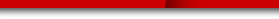# Аккорды и текст: Beatles - "Let it be"» Прицепы купить легковой прицеп avtopricep77.ru.
» Акция скидка на кузовной ремонт кузовной ремонт акции.

# Let it be

## Beatles

```
C G Am G F F^ C G F C/E Dm7 C
C               G                Am       G  F       F^
When i find miself in times of trouble mother Mary comes to me
C                  G            F C/E Dm7 C
Speaking words of wisdom,Let it be.
G               Am            G   F       F^
And in my hour of darkness she is standing right in front of me
C                 G             F C/E Dm7 c
Speaking words of wisdom,let it be.
AM         G          F          C                     G
Let it be, let it be, let it be, let it be, whisper words of wisdom,
F
let it be.

C/E Dm7 C

C                G              Am        G     F     F6
And when the broken - hearted people living in the world agree
C                G              F C/E Dm7 C
There will be an answer, let it be.
C                   G            Am             G     F        F6
For though they may be parted there is still a chance that they will see
C               G              F C/E Dm7 C
There will be an answer, let it be.
Am        G          F           C
be, let it be, let it be. let it be, yeah, there will be an answer,
G Let it
let   F C/E
Dm7 C
it be

G    Am         G          F          C                       G
Let it be, let it be, let it be, let it be, whisper words of wisdom,
F C/E Dm7 C F Em Dm7 C B F/A G F C F C G F C G Am G F F6 C G F C/E Dm7 C
Let it be.                                      (Guitar Solo)
Am         G          F          C                       G
Let it be, let it be, let it be, let it be, whisper words of wisdom,
FC/EDm7C
let it be.
C                  G              Am            G     F      F6
And when the night is cloudy there is still a light that shines on me
C            G              F C/E Dm7 C
Shine until tomorrow, Let it be.
C               G             Am       G    F         F6
I wake up to the sound of music Mother Mary comes to me
C                G             F C/E Dm7 C
Speaking words of wisdom, Let it be
Am          G          F                C                   G  F
Let it be, Let it be, Let it be, yeah, Let it be, yeah, there will be an
C/E Dm7 C
Am          G          F                C                    G
Let it be, Let it be, Let it be, yeah, Let it be, There will be an answer,
F
Let
C/E Dm7 C
it be.
Am          G          F                C
Let it be, Let it be, Let it be, yeah, Let it be
G             F C/E Dm7 C F Em Dm7 C B F/A G F C
Wisper words of wisdom, Let it be.

C              G
When I find myself in times of trouble
Am      G  Fmaj7   F
Mother Mary comes to me,
C                 G           F   C Dm7 C
Speaking words of wisdom,let it be
G
And in my hour of darkness
Am           G      F
She is standing right in front of me
C                 G            F  C Dm7 C
Speaking words of wisdom, let it be

G   Am7       G
Let it be, let it be
F        C
Let it be, let it be
G             F  C Dm7 C
Whisper words of wisdom, let it be

```